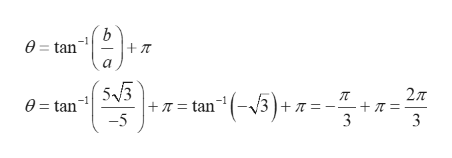# Graph the complex number, find its modulus and put the complex number n in polar form with arguement Θ and 2π.-5 + 5√3i

Question
67 views

Graph the complex number, find its modulus and put the complex number n in polar form with arguement Θ and 2π.

-5 + 5√3i

check_circle

Step 1

Given complex number z = -5 + 5√3i.

Graph the complex number.

Step 2

Find the modulus of complex number.

Step 3

Find the argument of complex number.

Since the complex n...help_outlineImage Transcriptionclosee= tan 5 3 + -5 27T л = tan"-/3)+7 = 0= tan -+ T = 3 3 fullscreen

### Want to see the full answer?

See Solution

#### Want to see this answer and more?

Solutions are written by subject experts who are available 24/7. Questions are typically answered within 1 hour.*

See Solution
*Response times may vary by subject and question.
Tagged in

### Algebra